# Solution Assignment 01 Hypothesis testing (one population)

1. Company blue-collar workers
The data for this assignment are listed in the Excel file Company blue-collar workers.
In the midst of labor-management negotiations, the president of a company argues that the company’s blue-collar workers, who are paid an average of 30000 dollars per year, are well-paid because the mean annual income of all blue-collar workers in the country is less than 30000 dollars. That figure is disputed by the union, which does not believe that the mean blue-collar income is less than 30000 dollars. To test the company president’s belief, an arbitrator draws a random sample of 350 blue-collar workers from across the country and asks each to report his or her annual income. The arbitrator assumes that the blue-collar incomes are normally distributed with a standard deviation of 8000 dollars.
a. Compute the mean blue-collar annual income of the sample.
b. What would you take as null hypothesis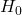and alternative hypothesis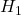?
c. What is the test statistic and can it be inferred at the 5% significance level that the company president is correct? Explain your answer.
d. What test statistic would you use if the population standard deviation of the blue-collar incomes is not known? Explain the consequences.

Solution.
a. The mean blue-collar income can be computed using Excel: 29120 dollars.
b.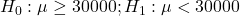c. The test statistic is: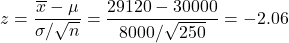.
This is a one-sided test and thus the rejection region is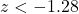, so there is not enough evidence to infer that the null hypothesis is correct. We may infer that the president is right. One could also compute the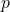-value which iswhich is much less than the significance level 0.05.
d. If the population standard deviation  would not be known, the sample standard deviation  is used. Then the test statistic would be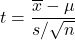. Instead of the rejection level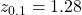the rejection level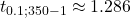has to be used. Since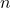is large the difference can be neglected.

0